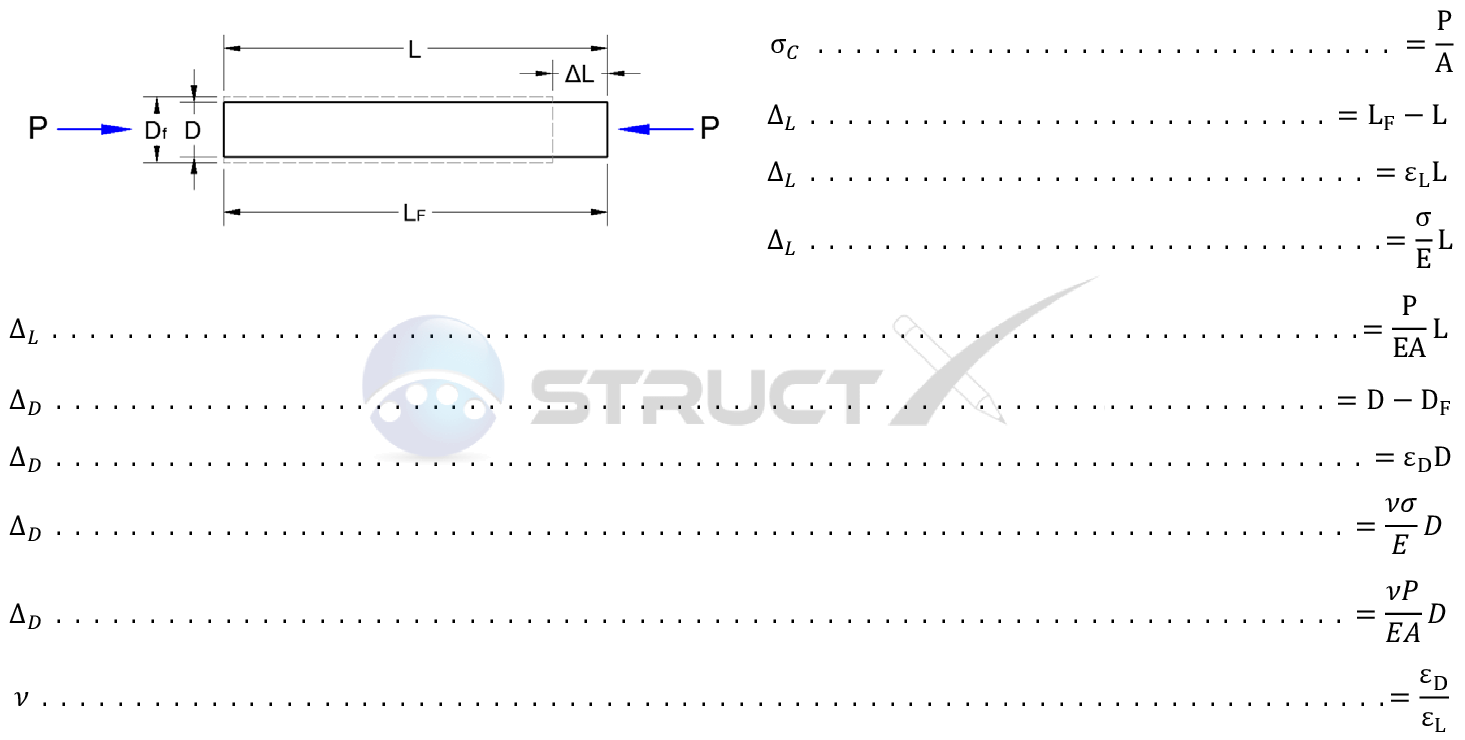# Compression Member - Change in DimensionsMore Forces# Notation and Units

## Metric and Imperial Units

The above formulas may be used with both imperial and metric units. As with all calculations care must be taken to keep consistent units throughout with examples of units which should be adopted listed below:

# Notation

• σc = compressive Stress, psi or MPa
• A = area, in4 or mm4
• D = depth, in or mm
• DF = final depth, in or mm
• E = modulus of elasticity, psi or MPa
• L = length under consideration, in or mm
• LF = final length, in or mm
• P = pressure under consideration, psi or MPa
• v = Poisson's ratio, unitless
• D = change in depth, in or mm
• L = change in length, in or mm
• σ = stress, psi or MPa
• ε = strain, unitless